Jet Set Go! All about Aeroplanes Jet Set Go! All about Aeroplanes

# Areas Related to Circles Class 10 Notes: Chapter 12

## CBSE Class 10 Maths Areas Related To Circles Notes:-

Get the complete notes on an area related to circles for class 10 is provided here. The concepts related to circles such as area, circumference, segment, sector, angle and length of a circle, area for the sector of a circle are provided here. Also, the visualization of some plane and solid figures areas are discussed here.

Students can refer to the short notes and MCQ questions along with separate solution pdf of this chapter for quick revision from the links below:

• Areas related to circles Short Notes
• Areas related to circles MCQ Practice Questions
• Areas related to circles MCQ Practice Solutions
• ## Introduction to Areas related to Circles

In chapter “Areas related to circles” for Class 10, we will learn to find the areas of circles, areas of segment and sector of circle, circumference, length of arc of a sector, etc. A circle is a two-dimensional figure. It is a curved-shape that has all its points at an equal distance from the center. Let us learn now how to find areas related to circle in this article.## Area of a Circle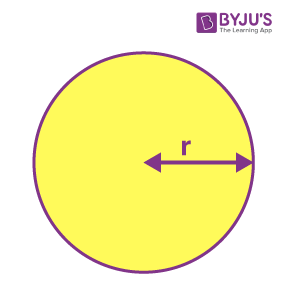Area of a circle is πr2, where π=22/7 or ≈ 3.14 (can be used interchangeably for problem-solving purposes) and r is the radius of the circle.
π is the ratio of the circumference of a circle to its diameter.
Example: Find the area of a circle with radius = 7cm.
Solution: Given, radius of circle = 7cm
By the formula we know;
Area of circle = πr2
= π(7)2
= (22/7) (7)2
= 154 sq.cm.

#### For More Information On Area Of Circle, Watch The Below Video.To know more about Area of a Circle, visit here.

## Circumference of a Circle

The circumference of a circle is the distance covered by going around its boundary once.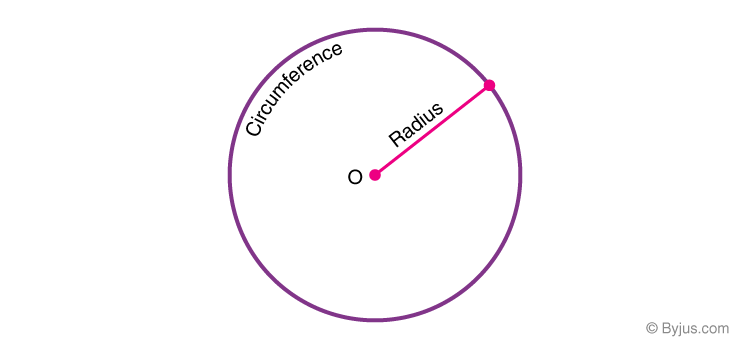The perimeter of a circle has a special name: Circumference, which is π times the diameter which is given by the formula;
Circumference of a circle = 2πr.

Example: The circumference of a circle whose radius is 21cm, is given by;
C = 2πr
= 2 (22/7) (21)
= 132 cm

To know more about Circumference of a Circle, visit here.

## Segment of a Circle

A circular segment is a region of a circle that is “cut off” from the rest of the circle by a secant or a chord.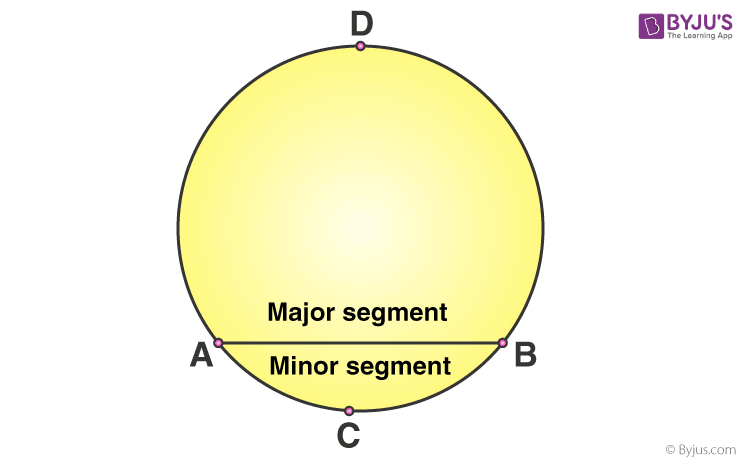To know more about Segment of a Circle, visit here.

## Sector of a Circle

A circle sector/ sector of a circle is defined as the region of a circle enclosed by an arc and two radii. The smaller area is called the minor sector and the larger area is called the major sector.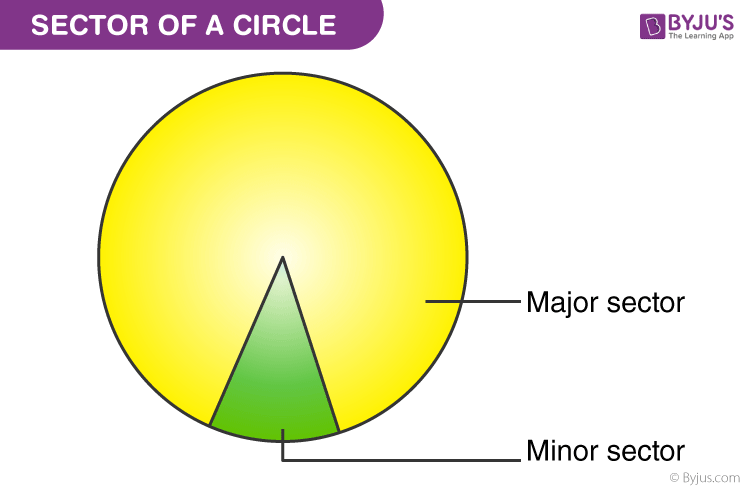#### For More Information On Parts Of A Circle, Watch The Below Video.To know more about Sector of a Circle, visit here.

### Angle of a Sector

The angle of a sector is the angle that is enclosed between the two radii of the sector.

### Area of a Sector of a Circle

Area of a sector is given by

(θ/360°)×πr2

where θ is the angle of this sector(minor sector in the following case) and r is its radius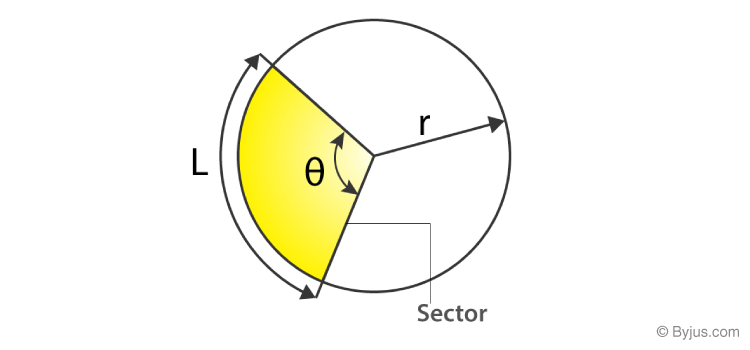Area of a sector

Example: Suppose the sector of a circle is 45° and radius is 4 cm, then the area of the sector will be:
Area = (θ/360°) × πr2

= (45°/360°) × (22/7) × 4 × 4

= 44/7 sq. cm
To know more about Sector of a Circle, visit here.

### Length of an arc of a sector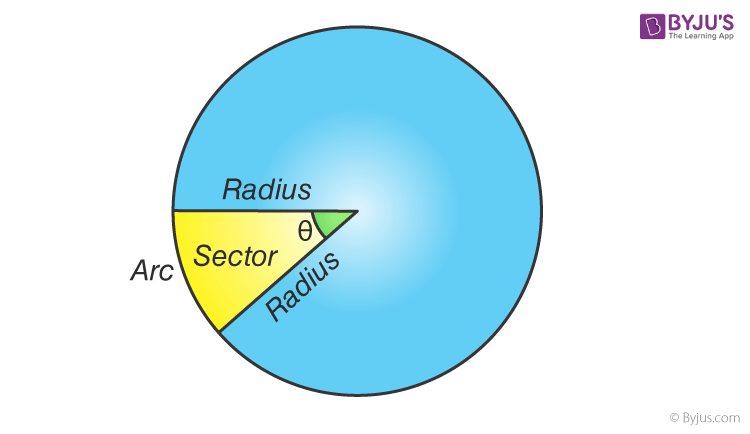The length of the arc of a sector can be found by using the expression for the circumference of a circle and the angle of the sector, using the following formula:

L= (θ/360°)×2πr

Where θ is the angle of sector and r is the radius of the circle.

### Area of a Triangle

The Area of a triangle is,
Area=(1/2)×base×height
If the triangle is an equilateral then
Area=(√3/4)×a2  where “a” is the side length of the triangle.

To know more about Area of a Triangle, visit here.

### Area of a Segment of a Circle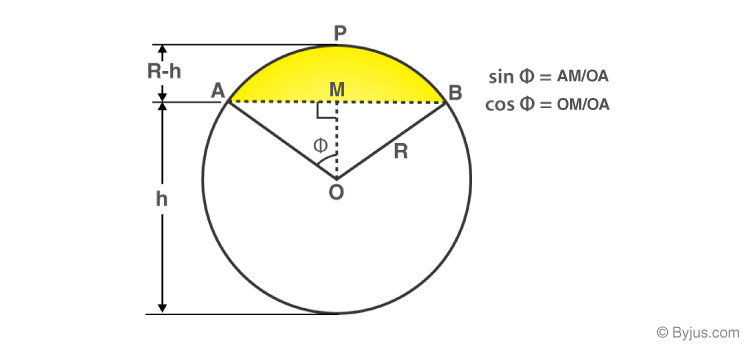Area of segment APB (highlighted in yellow)
= (Area of sector OAPB) – (Area of triangle AOB)

=[(∅/360°)×πr2] – [(1/2)×AB×OM]

[To find the area of triangle AOB, use trigonometric ratios to find OM (height) and AB (base)]

Also, the Area of segment APB can be calculated directly if the angle of the sector is known using the following formula.

=[(θ/360°)×πr2] – [r2×sin θ/2 × cosθ/2]

Where θ is the angle of the sector and r is the radius of the circle.

#### Formulas List

All these formulas are tabulated as given below for quick revision.

 Parameters of Circles Formulas Area of the sector of angle θ (θ/360°) × πr2 Length of an arc of a sector of angle θ (θ/360°) × 2πr Area of major sector πr2 – (θ/360°) × πr2 Area of a segment of a circle Area of the corresponding sector – Area of the corresponding triangle Area of the major segment πr2 – Area of segment (minor segment)

## Visualizations

### Areas of different plane figures

–    Area of a square (side l) =l2
–    Area of a rectangle =l×b, where l and b are the length and breadth of the rectangle
–    Area of a parallelogram =b×h, where “b” is the base and “h” is the perpendicular height.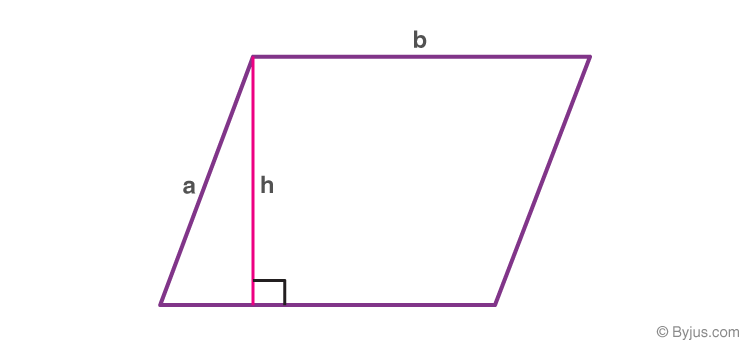parallelogram

Area of a trapezium =[(a+b)×h]/2,

where

a & b are the length of the parallel sides

h is the trapezium height

Area of a rhombus =pq/2, where p & q are the diagonals.

#### For More Information On Visualizing Areas of different plane figures, Watch The Below Video.To know more about Areas of different plane figures, visit here.

### Areas of Combination of Plane figures

For example: Find the area of the shaded part in the following figure: Given the ABCD is a square of side 28 cm and has four equal circles enclosed within.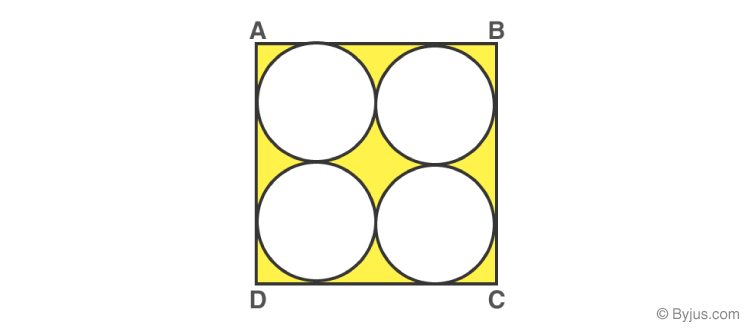Looking at the figure we can visualize that the required shaded area = A(square ABCD)  4 ×A(Circle).

Also, the diameter of each circle is 14 cm.
=(l2)4×(πr2)
=(282)[4×(π×49)]
=784[4×22/7×49]
=784616
=168cm2

### Practice Questions

1. Find the area of the sector of a circle with a radius of 4 cm and of angle 30°. Also, find the area of the corresponding major sector (Use π = 3.14).
2. A chord of a circle of radius 15 cm subtends an angle of 60° at the centre. Find the areas of the corresponding minor and major segments of the circle. (Use π = 3.14 and 3 = 1.73)
3. To warn ships of underwater rocks, a lighthouse spreads a red-coloured light over a sector of angle 80° to a distance of 16.5 km. Find the area of the sea over which the ships are warned. (Use π = 3.14)